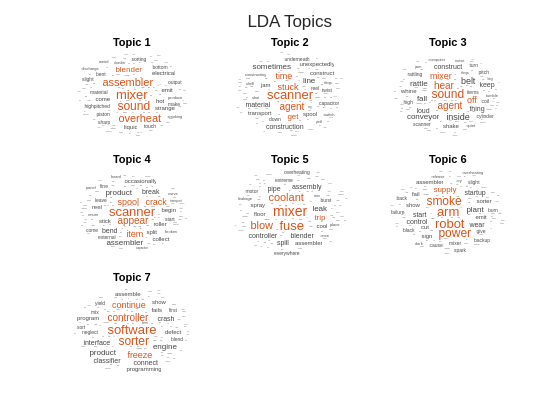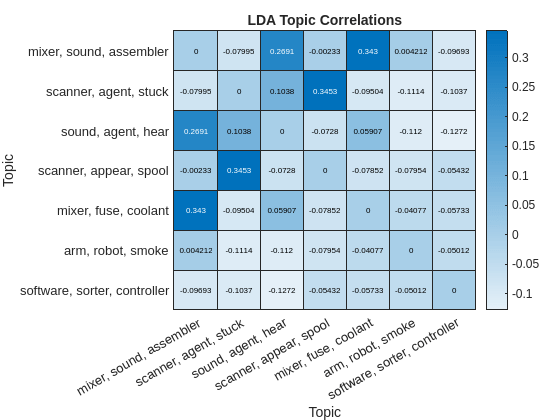# Visualize LDA Topic Correlations

This example shows how to analyze correlations between topics in a Latent Dirichlet Allocation (LDA) topic model.

A latent Dirichlet allocation (LDA) model is a topic model which discovers underlying topics in a collection of documents and infers word probabilities in topics. The vectors of per-topic word probabilities characterize the topics. Using the per-topic word probabilities, you can identify correlations between the topics.

Load the LDA model `factoryReportsLDAModel` which is trained using a data set of factory reports detailing different failure events. For an example showing how to fit an LDA model to a collection of text data, see Analyze Text Data Using Topic Models.

```load factoryReportsLDAModel mdl```
```mdl = ldaModel with properties: NumTopics: 7 WordConcentration: 1 TopicConcentration: 0.5755 CorpusTopicProbabilities: [0.1587 0.1573 0.1551 0.1534 0.1340 ... ] DocumentTopicProbabilities: [480x7 double] TopicWordProbabilities: [158x7 double] Vocabulary: ["item" "occasionally" "get" ... ] TopicOrder: 'initial-fit-probability' FitInfo: [1x1 struct] ```

Visualize the topics using word clouds.

```numTopics = mdl.NumTopics; figure t = tiledlayout("flow"); title(t,"LDA Topics") for i = 1:numTopics nexttile wordcloud(mdl,i); title("Topic " + i) end```### Visualize Topic Correlations

Calculate the correlations between the topics using the `corrcoef` function with the LDA model topic word probabilities as input.

`correlation = corrcoef(mdl.TopicWordProbabilities);`

View the correlations in a heat map and label each topic with its top three words. To prevent the heat map from highlighting the trivial correlations between topics each and itself, subtract the identity matrix from the correlations.

For each topic, find the top three words.

```numTopics = mdl.NumTopics; for i = 1:numTopics top = topkwords(mdl,3,i); topWords(i) = join(top.Word,", "); end```

Plot the correlations using the `heatmap` function.

```figure heatmap(correlation - eye(numTopics), ... XDisplayLabels=topWords, ... YDisplayLabels=topWords) title("LDA Topic Correlations") xlabel("Topic") ylabel("Topic")```For each topic, find the topic with the strongest correlation and display the pairs in a table with the corresponding correlation coefficient.

```[topCorrelations,topCorrelatedTopics] = max(correlation - eye(numTopics)); tbl = table; tbl.TopicIndex = (1:numTopics)'; tbl.Topic = topWords'; tbl.TopCorrelatedTopicIndex = topCorrelatedTopics'; tbl.TopCorrelatedTopic = topWords(topCorrelatedTopics)'; tbl.CorrelationCoefficient = topCorrelations'```
```tbl=7×5 table TopicIndex Topic TopCorrelatedTopicIndex TopCorrelatedTopic CorrelationCoefficient __________ ______________________________ _______________________ ______________________________ ______________________ 1 "mixer, sound, assembler" 5 "mixer, fuse, coolant" 0.34304 2 "scanner, agent, stuck" 4 "scanner, appear, spool" 0.34526 3 "sound, agent, hear" 1 "mixer, sound, assembler" 0.26909 4 "scanner, appear, spool" 2 "scanner, agent, stuck" 0.34526 5 "mixer, fuse, coolant" 1 "mixer, sound, assembler" 0.34304 6 "arm, robot, smoke" 1 "mixer, sound, assembler" 0.0042125 7 "software, sorter, controller" 7 "software, sorter, controller" 0 ```